# ML Aggarwal Class 8 Solutions for ICSE Maths Chapter 6 Operation on Sets Venn Diagrams Ex 6.2

## ML Aggarwal Class 8 Solutions for ICSE Maths Chapter 6 Operation on Sets Venn Diagrams Ex 6.2

Question 1.
From the adjoining Venn diagram, find the following sets :
(i) A
(ii) B
(iii) ξ
(iv) A’
(v) B’
(vi) A ∪ B
(vii) A ∩ B
(viii) (A ∪ B)’
(ix) (AB)’
Solution:Question 2.
From the adjoining Venn diagram, find the following sets :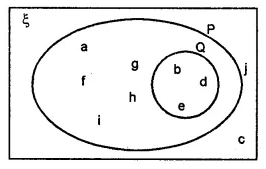(i) P
(ii) Q
(iii) ξ
(iv) P’
(v) Q’
(vi) P ∪ Q
(vii) P ∩ Q
(viii) (P ∪ Q)’
(ix) (P ∩ Q)’
Solution: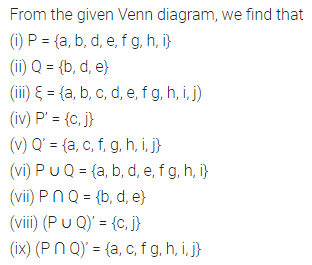Question 3.
From the adjoining Venn diagram, find the following sets :
(i) ξ
(ii) A ∩ B
(iii) A ∩ B ∩ C
(iv) C’
(v) A – C
(vi) B – C
(vii) C – B
(viii) (A ∪ B)’
(ix) (A ∪ B ∪ C)’Solution:Question 4.
Draw Venn diagrams to show the relationship between the following pairs of sets :
(i) A = {x | x ϵ N, x = 2n, n ≤ 5} and
B = {x | x ϵ W, x = 4n, n < 5}
(ii) A = {prime factors of 42} and
B = {prime factors of 60}
(iii) P = {x | x ϵ W, x < 10} and
Q = {prime factors of 210}
Solution: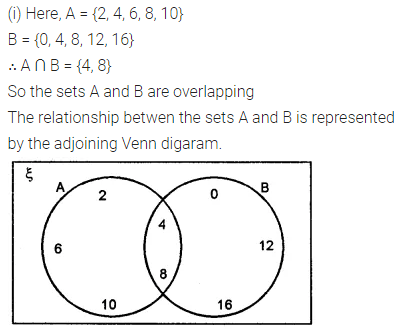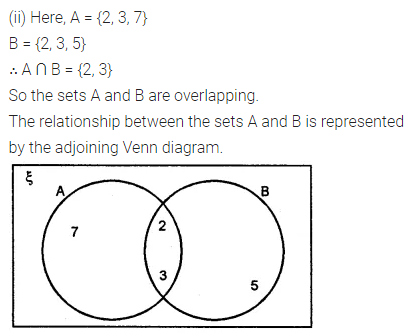Question 5.
Draw a Venn diagram to illustrate the following information:
n (A) = 22, n (B) = 18 and n (A ∩ B) = 5.
Hence find:
(i) n (A ∩ B)
(ii) n (A – B)
(iii) n (B – A)
Solution: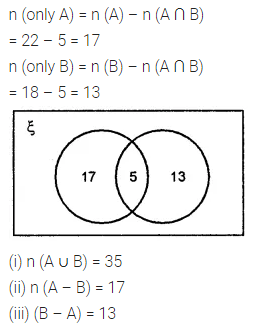Question 6.
Draw a Venn diagram to illustrate the following information: n (A) = 25, n (B) = 16, n (A ∩ B) = 6 and n ((A ∪ B)’) = 5
Hence find:
(i) n (A ∪ B)
(ii) n (ξ)
(iii) n (A – B)
(iv) n (B – A)
Solution:Question 7.
Given n(ξ) = 25, n (A’) = 7, n (B) = 10 and B ⊂ A. Draw a Venn diagram to illustrate this information. Hence, find the cardinal number of the set A – B.
Solution:Question 8.
In a group of 50 boys, 20 play only cricket, 12 play only football and 5 boys play both the games. Draw a Venn diagram and find the number of boys who play
(i) at least one of the two games cricket or football.
(ii) neither cricket nor football.
Solution: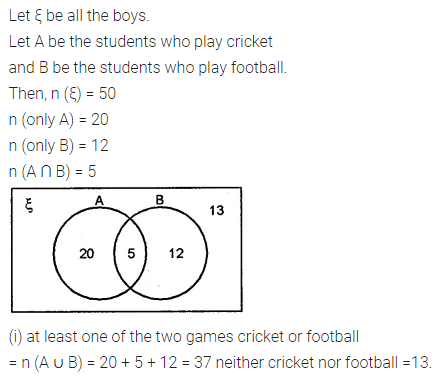Question 9.
In a group of 40 students, 26 students like orange but not banana, while 32 students like orange. If all the students like atleast one of the two fruits, find the number of students who like
(i) both orange and banana
(ii) only banana.
Draw a Venn diagram to represent the data.
Solution: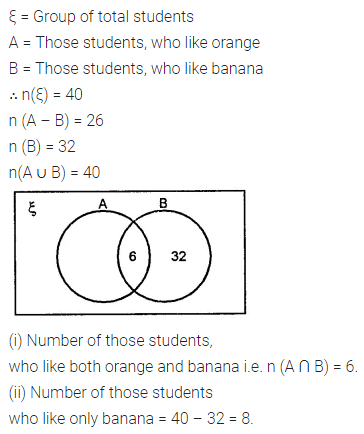Question 10.
In a group of 60 persons, 45 speak Bengali, 28 speak English and all the persons speak atleast one language. Find how many people speak both Bengali and English. Draw a Venn diagram.
Solution: